the Pythagorean theorem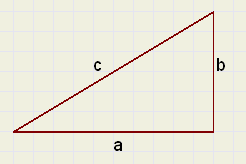Introduction
In a triangle with rectangle sides of length a en b and hypothenuse c
the equation :

a2 + b2 = c2

is true.

This equation is known as the Pythagoras theorem.
See picture at right.

This theorem is the basis of many other theorems and calculations.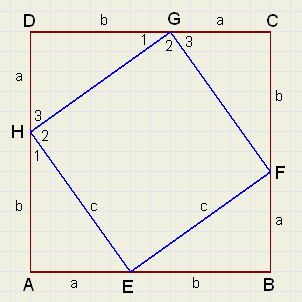Proof -1-
On the sides, points E,F,G,H are placed such that AE = BF = CG = DH.
We call this distance a.
A side has length a + b.

The proof has two parts:
1. Proof that EFGH is a square.
The construction implies that triangles AEH,BFE,CGF and DHG
are congruent.
So, in any case, EFGH is a rhombus.
The length of a side we call c.
Angles (1) and (3) are 90 degrees together, so angle (2) is 90 degrees.
EFGH is a square.

2. the equation:
Let's write an area as [ ]
Look at the areas and notice that:

[ABCD] = 4 * [AEH] + [EFGH]  (a + b)2 = 2ab + c2 a2 + 2ab + b2 = 2ab + c2 a2 + b2 = c2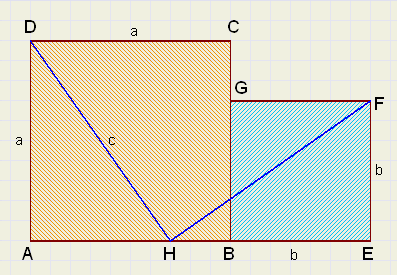Proof -2-
ABCD with a side of a
BEFG with a side of b.
Point H is on AB such, that AH = b.
Which implies that HE = a.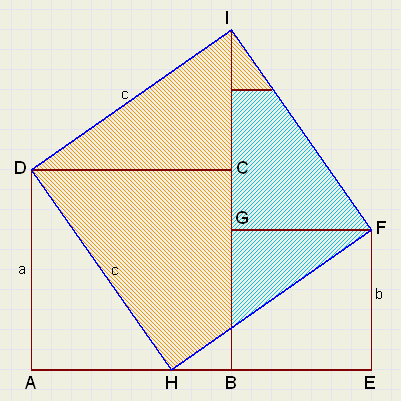We use the scissors and cut triangles ADH and HEF
placing them on sides DC and GF.

Similar to proof -1- we see, that HFID is a square.

The coloring shows:
 a2 + b2 = c2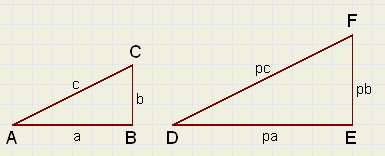Proof -3-
First a preamble.
Right are pictured two triangles: ABC and DEF.
These triangles are similar:
One is an enlargement of the other, the multiplication factor is p.

Notice the similar sides: AB,DE, or AC,DF.
Similar triangles have the properties:
- similar angles are equal
- ratio's of similar sides are equal
Conversely: two triangles are similar if their angles are equal.

Lemma

The areas of similar triangles have a ratio equal to the square of ratio's of similar sides
The proof unfolds: (see figure above)
Double the area of the triangles is ab and abp2.
The ratio of areas is 1 : p2 which is the ratio of similar sides, power 2.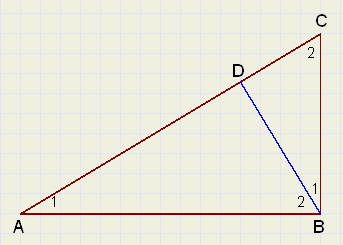Picture right has BD perpendicular to AC and AB perpendicular to BC.
There are three similar triangles: ABC, ADB and BDC.
Note the correct sequence of the angles for similarity.
Equal angles are marked equally.

With the previous knowledge we may proceed:

(call AB = a , BC = b , AC = c and notice similar hypothenuses)

We see:
[ADB] : [ABC] + [ABC] : [ABC] = 1

and because of the lemma:
 a2 : c2 + b2 : c2 = 1 a2 + b2 = c2
Proof -4-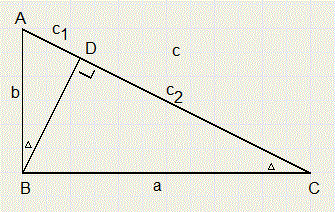Here we make use of similarity as well.

1.
DABD ~DBCD
c1 : b = b : c
c1c = b2
2.
DBCD ~DACB
c2 : a = a : c
c2c = a2